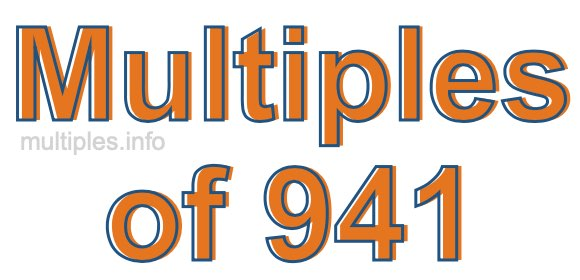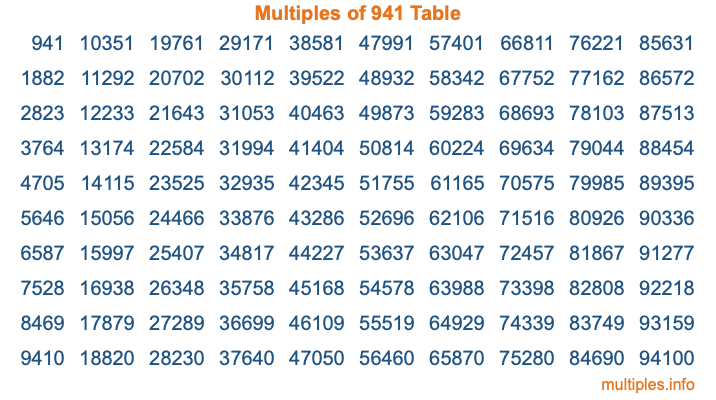Multiples of 941Welcome to the Multiples of 941 page. Here we will first teach you everything you will ever need to know about the multiples of 941, and then give you a study guide summary of everything we taught you to make sure you remember it all. Use this page to look up facts and learn information about the multiples of 941. This page will make you a multiples of nine hundred forty-one expert!

Definition of Multiples of 941
Multiples of 941 are all the numbers that when divided by 941 equal an integer. Each of the multiples of 941 are called a multiple. A multiple of 941 is created by multiplying 941 by an integer.

Therefore, to create a list of multiples of 941, you start with 1 multiplied by 941, then 2 multiplied by 941, then 3 multiplied by 941, and so on for as long as you want. Thus, the list of the first five multiples of 941 is 941, 1882, 2823, 3764, and 4705. To see a larger list of multiples of 941, see the printable image of Multiples of 941 further down on this page. We also have a category where you can choose any nth multiple of 941.

Multiples of 941 Checker
The Multiples of 941 Checker below checks to see if any number of your choice is a multiple of 941. In other words, it checks to see if there is any number (integer) that when multiplied by 941 will equal your number. To do that, we divide your number by 941. If the the quotient is an integer, then your number is a multiple of 941.

Is  a multiple of 941?

Least Common Multiple of 941 and ...
A Least Common Multiple (LCM) is the lowest multiple that two or more numbers have in common. This is also called the smallest common multiple or lowest common multiple and is useful to know when you are adding our subtracting fractions. Enter one or more numbers below (941 is already entered) to find the LCM.

Check out our LCM Calculator if you need more details about the Least Common Multiple or if you need the LCM for different numbers for adding and subtraction fractions.

nth Multiple of 941
As we stated above, 941 is the first multiple of 941, 1882 is the second multiple of 941, 2823 is the third multiple of 941, and so on. Enter a number below to find the nth multiple of 941.

th multiple of 941

Multiples of 941 vs Factors of 941
941 is a multiple of 941 and a factor of 941, but that is where the similarities end. All postive multiples of 941 are 941 or greater than 941. All positive factors of 941 are 941 or less than 941.

Below is the beginning list of multiples of 941 and the factors of 941 so you can compare:

Multiples of 941: 941, 1882, 2823, 3764, 4705, etc.

Factors of 941: 1, 941

As you can see, the multiples of 941 are all the numbers that you can divide by 941 to get a whole number. The factors of 941, on the other hand, are all the whole numbers that you can multiply by another whole number to get 941.

It's also interesting to note that if a number (x) is a factor of 941, then 941 will also be a multiple of that number (x).

Multiples of 941 vs Divisors of 941
The divisors of 941 are all the integers that 941 can be divided by evenly. Below is a list of the divisors of 941.

Divisors of 941: 1, 941

The interesting thing to note here is that if you take any multiple of 941 and divide it by a divisor of 941, you will see that the quotient is an integer.

Multiples of 941 Table
Below is an image of the first 100 multiples of 941 in a table. The table is in chronological order, column by column. The first column has the first ten multiples of 941, the second column has the next ten multiples of 941, and so on.The Multiples of 941 Table is also referred to as the 941 Times Table or Times Table of 941. You are welcome to print out our table for your studies.

Negative Multiples of 941
Although not often discussed or needed in math, it is worth mentioning that you can make a list of negative multiples of 941 by multiplying 941 by -1, then by -2, then by -3, and so on, to get the following list of negative multiples of 941:

-941, -1882, -2823, -3764, -4705, etc.

Multiples of 941 Summary
Below is a summary of important Multiples of 941 facts that we have discussed on this page. To retain the knowledge on this page, we recommend that you read through the summary and explain to yourself or a study partner why they hold true.

There are an infinite number of multiples of 941.

A multiple of 941 divided by 941 will equal a whole number.

941 divided by a factor of 941 equals a divisor of 941.

The nth multiple of 941 is n times 941.

The largest factor of 941 is equal to the first positive multiple of 941.

941 is a multiple of every factor of 941.

941 is a multiple of 941.

A multiple of 941 divided by a divisor of 941 equals an integer.

941 divided by a divisor of 941 equals a factor of 941.

Any integer times 941 will equal a multiple of 941.

Multiples of a Number
Here you can get the multiples of another number, all with the same attention to detail as we did for multiples of 941 on this page.

Multiples of
Multiples of 942
Did you find our page about multiples of nine hundred forty-one educational? Do you want more knowledge? Check out the multiples of the next number on our list!